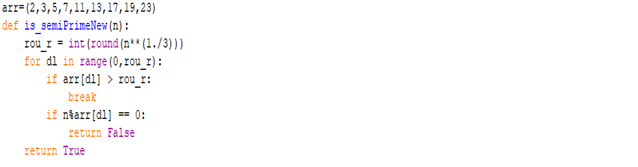## Below is my first discovery in the world of prime numbers:

Problem statement
A semi prime (also called biprime or 2-almost prime, or pq number) is a natural number that is the product of two (not necessarily distinct) prime numbers(Source:Wikipedia)
The only way to check whether a given number is prime with 100% probability is finding whether the given number has factors when you iterate between the value “2” and square root of the given number.
The same number of iterations are used to determine whether a given number is semi-prime.
Solution
With 100% probability we can check whether a given number is prime/semi-prime, with very very less time complexity.
Below is the process to follow:
1.       Find the cube root of the given number(let’s say this as “n”). Let’s say the cube root is “cn”
2.       Make a list of all the prime numbers till the prime number which is greater than “cn”, but is the least. So if “cn” is “4”, we need to take prime numbers till “5”.
3.       Check any of the prime number in step-2 divides “n”. If yes, then the “n” is neither prime nor semi-prime
4.       If we exhaust all the prime numbers in step-2 and still nothing divides “n”, then “n” is prime or semi-prime
Example
Let’s say we take any number below 10,000 and want to confirm whether it is prime/semi-prime/none.
Cuberoot of 10,000 is 21.544. Rounding off to nearest integer it is 22.
Take the prime numbers till 23(which is the least prime number that is greater than cuberoot of number we want to determine) and see if any of these divides our number. If nothing is able to divide then our number is prime/semi-prime.In python the implementation will be as below, if we consider first 10,000 positive integers(note the array is initialized to first 9 prime numbers). Any of the first 10,000 positive integers can be evaluated to be prime/semi-prime using the below function. For numbers greater than 10,000 we just need to increment “arr” array.
Impact
Below are some of the possible applications for this solution:
1.       To check whether a given number is prime/not requires exponential time complexity as the number grows big. Although our solution results in Semi-primes along with primes, this helps in narrowing down quickly whether the number is not a prime.
2.       Semi-primes are used in RSA algorithm where the multiple of two large primes is used for modulus.
3.       Other applications that use large prime numbers in cyber security

Compared this with miller-rabin test with first 50,000 positive integers and the results generated are the same. Used 40 iterations for miller-rabin test which is minimum required to get good probability for Miller-rabin.
Although the results generated are same with Miller-rabin, the time taken to check whether the given number is prime/Semi-prime and print them with my solution is nearly half. Below are the times in a 1.6 GHZ clockspeed Ubuntu M/c.
 Miller-Rabin(In seconds) Proposed Solution(In Seconds) Real 0.497 0.227 User 0.188 0.183 Sys 0.026 0.025